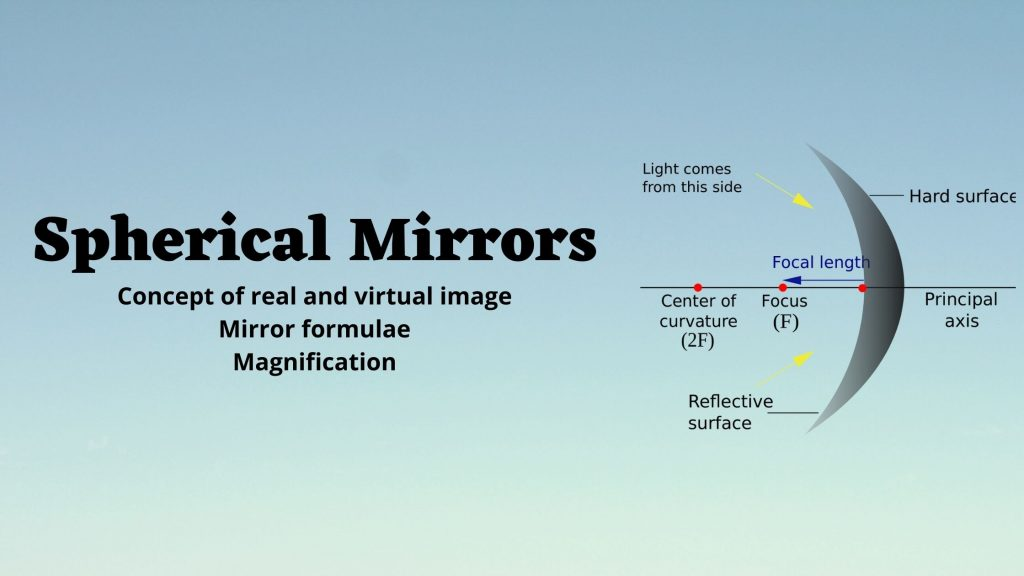Physics
Study Material

# Spherical Mirrors- Formula, Magnification, Real And Virtual Image

3 minutes long
Posted by Mahak Jain, 11/8/2021Hesap Oluştur

Got stuck on homework? Get your step-by-step solutions from real tutors in minutes! 24/7. Unlimited.

We are familiar with the laws of reflection. The angle of reflection (i.e., the angle between reflected ray and the normal to the reflecting surface or the mirror) equals the angle of incidence (angle between incident ray and the normal). Also that the incident ray, reflected ray and the normal to the reflecting surface at the point of incidence lie in the same plane (Fig. 9.1). These laws are valid at each point on any reflecting surface whether plane or curved. However, we shall restrict our discussion to the special case of curved surfaces, that is, spherical surfaces.

The normal in this case is to be taken as normal to the tangent to surface at the point of incidence. That is, the normal is along the radius, the line joining the centre of curvature of the mirror to the point of incidence. We have already studied that the geometric centre of a spherical mirror is called its pole while that of a spherical lens is called its optical centre. The line joining the pole and the centre of curvature of the spherical mirror is known as the principal axis. In the case of spherical lenses, the principal axis is the line joining the optical centre with its principal focus as you will see later.

## Sign convention

To derive the relevant formulae for reflection by spherical mirrors and refraction by spherical lenses, we must first adopt a sign convention for measuring distances. In this book, we shall follow the Cartesian sign convention. According to this convention, all distances are measured from the pole of the mirror or the optical centre of the lens. The distances measured in the same direction as the incident light are taken as positive and those measured in the direction opposite to the direction of incident light are taken as negative (Fig. 9.2). The heights measured upwards with respect to x-axis and normal to the principal axis (x-axis) of the mirror/ lens are taken as positive (Fig. 9.2). The heights measured downwards are taken as negative.

## Focal length of spherical mirrors

We assume that the rays are paraxial, i.e., they are incident at points close to the pole P of the mirror and make small angles with the principal axis. The reflected rays converge at a point F on the principal axis of a concave mirror [Fig. 9.3(a)]. For a convex mirror, the reflected rays appear to diverge from a point F on its principal axis [Fig. 9.3(b)]. The point F is called the principal focus of the mirror. If the parallel paraxial beam of light were incident, making some angle with the principal axis, the reflected rays would converge (or appear to diverge) from a point in a plane through F normal to the principal axis. This is called the focal plane of the mirror.